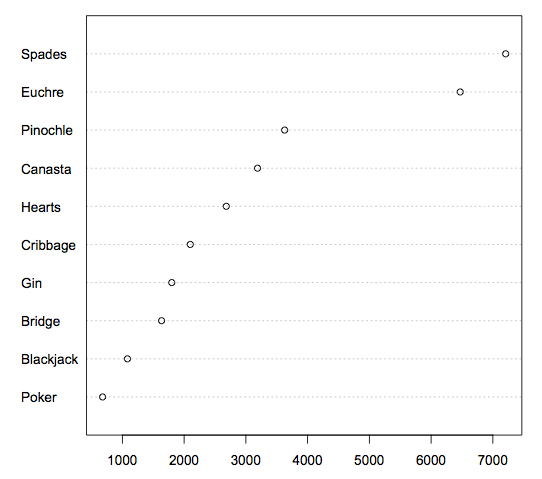# 2.10: Dot Plots

•• Contributed by David Lane
• Associate Professor (Psychology, Statistics, and Management) at Rice University

Skills to Develop

• Create and interpret dot plots
• Judge whether a dot plot would be appropriate for a given data set

Dot plots can be used to display various types of information. Figure $$\PageIndex{1}$$ uses a dot plot to display the number of M & M's of each color found in a bag of M & M's. Each dot represents a single M & M. From the figure, you can see that there were $$3$$ blue M & M's, $$19$$ brown M & M's, etc.Figure $$\PageIndex{1}$$: A dot plot showing the number of M & M's of various colors in a bag of M & M's

The dot plot in Figure $$\PageIndex{2}$$ shows the number of people playing various card games on the Yahoo website on a Wednesday. Unlike Figure $$\PageIndex{1}$$, the location rather than the number of dots represents the frequency.Figure $$\PageIndex{2}$$: A dot plot showing the number of people playing various card games on a Wednesday

The dot plot in Figure $$\PageIndex{3}$$ shows the number of people playing on a Sunday and on a Wednesday. This graph makes it easy to compare the popularity of the games separately for the two days, but does not make it easy to compare the popularity of a given game on the two days.Figure $$\PageIndex{3}$$: A dot plot showing the number of people playing various card games on a Sunday and on a WednesdayFigure $$\PageIndex{4}$$: An alternate way of showing the number of people playing various card games on a Sunday and on a Wednesday

The dot plot in Figure $$\PageIndex{4}$$ makes it easy to compare the days of the week for specific games while still portraying differences among games.

## Contributors

• Online Statistics Education: A Multimedia Course of Study (http://onlinestatbook.com/). Project Leader: David M. Lane, Rice University.